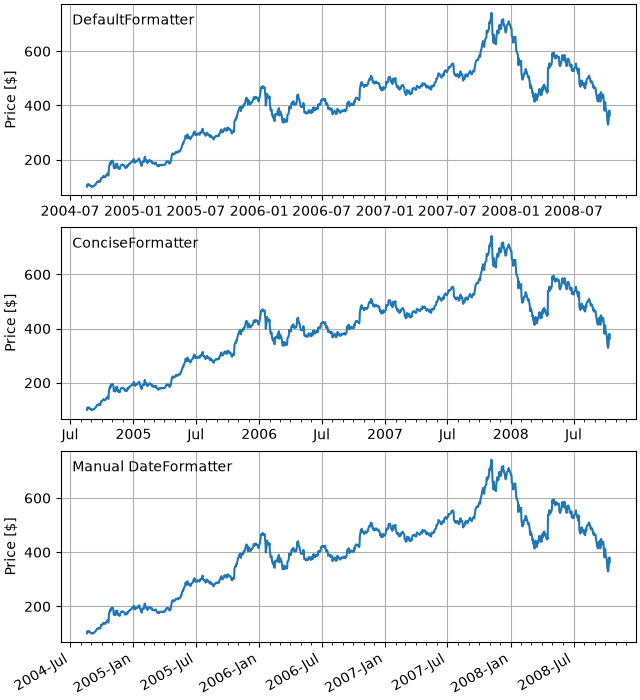# Date tick labels¶

Show how to make date plots in Matplotlib using date tick locators and formatters. See Major and minor ticks for more information on controlling major and minor ticks.

Matplotlib date plotting is done by converting date instances into days since an epoch (by default 1970-01-01T00:00:00). The matplotlib.dates module provides the converter functions date2num and num2date, which convert datetime.datetime and numpy.datetime64 objects to and from Matplotlib's internal representation. These data types are registered with with the unit conversion mechanism described in matplotlib.units, so the conversion happens automatically for the user. The registration process also sets the default tick locator and formatter for the axis to be AutoDateLocator and AutoDateFormatter. These can be changed manually with Axis.set_major_locator and Axis.set_major_formatter; see for example Date Demo Convert.

An alternative formatter is the ConciseDateFormatter as described at Formatting date ticks using ConciseDateFormatter, which often removes the need to rotate the tick labels.import numpy as np
import matplotlib.pyplot as plt
import matplotlib.dates as mdates
import matplotlib.cbook as cbook

# Load a numpy structured array from yahoo csv data with fields date, open,
# close, volume, adj_close from the mpl-data/example directory.  This array
# stores the date as an np.datetime64 with a day unit ('D') in the 'date'
# column.

fig, ax = plt.subplots()

# Major ticks every 6 months.
fmt_half_year = mdates.MonthLocator(interval=6)
ax.xaxis.set_major_locator(fmt_half_year)

# Minor ticks every month.
fmt_month = mdates.MonthLocator()
ax.xaxis.set_minor_locator(fmt_month)

# Text in the x axis will be displayed in 'YYYY-mm' format.
ax.xaxis.set_major_formatter(mdates.DateFormatter('%Y-%m'))

# Round to nearest years.
datemin = np.datetime64(data['date'], 'Y')
datemax = np.datetime64(data['date'][-1], 'Y') + np.timedelta64(1, 'Y')
ax.set_xlim(datemin, datemax)

# Format the coords message box, i.e. the numbers displayed as the cursor moves
# across the axes within the interactive GUI.
ax.format_xdata = mdates.DateFormatter('%Y-%m')
ax.format_ydata = lambda x: f'\${x:.2f}'  # Format the price.
ax.grid(True)

# Rotates and right aligns the x labels, and moves the bottom of the
# axes up to make room for them.
fig.autofmt_xdate()

plt.show()


Keywords: matplotlib code example, codex, python plot, pyplot Gallery generated by Sphinx-Gallery